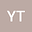•One of the fundamental research problems in the theory of generalized inverses of matrices is to establish reverse order laws for generalized inverses of matrix products. Under the assumption that $A$, $B$, and $C$ singular matrices of the appropriate sizes, two reverse order laws for generalized inverses of the matrix products $AB$ and $ABC$ can be written as $(AB)^{(i,\ldots,j)} = B^{(i_2,\ldots,j_2)}A^{(i_1,\ldots,j_1)}$ and $(ABC)^{(i,\ldots,j)} = C^{(i_3,\ldots,j_3)}B^{(i_2,\ldots,j_2)}A^{(i_1,\ldots,j_1)}$, or other mixed reverse order laws. These equalities do not necessarily hold for different choices of generalized inverses of the matrices. Thus it is a tremendous work to classify and derive necessary and sufficient conditions for the reverse order law to hold because there are all 15 types of $\{i,\ldots, j\}$-generalized inverse for a given matrix according to combinatoric choices of the four Penrose equations. In this paper, we shall establish several decades of mixed reverse order laws for $\{1\}$- and $\{1,2\}$-generalized inverses of $AB$ and $ABC$, and give a classified investigation to a family of reverse order laws $(ABC)^{(i,\ldots,j)} = C^{-1}B^{(k,\ldots,l)}A^{-1}$ for the eight commonly-used types of generalized inverses by means of the block matrix representation method (BMRM) and the matrix rank method (MRM). A variety of consequences and applications these reverse order laws are presented.